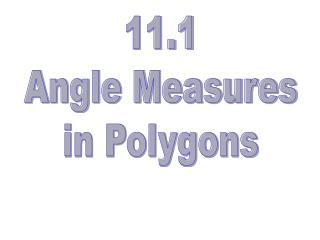DownloadDownload Presentation11.1 Angle Measures in Polygons

# 11.1 Angle Measures in Polygons

Télécharger la présentation## 11.1 Angle Measures in Polygons

- - - - - - - - - - - - - - - - - - - - - - - - - - - E N D - - - - - - - - - - - - - - - - - - - - - - - - - - -
##### Presentation Transcript

1. 11.1 Angle Measures in Polygons

2. Sum of measures of interior angles # of triangles # of sides 1(180)=180 3 1 2(180)=360 4 2 3 3(180)=540 5 6 4 4(180)=720 n-2 (n-2) • 180 n

3. If a convex polygon has n sides, then the sum of the measure of the interior angles is (n – 2)(180°)

4. If a regular convex polygon has n sides, then the measure of one of the interior angles is

5. Ex. 1 Use a regular 15-gon to answer the questions. • Find the sum of the measures of the interior angles. • Find the measure of ONE interior angle 2340° 156°

6. Ex: 2 Find the value of x in the polygon x 126 100 143 130 117 126 + 130 + 117 + 143 + 100 + x = 720 616 + x = 720 x = 104

7. Ex: 3 The measure of each interior angle is 150°, how many sides does the regular polygon have? One interior angle A regular dodecagon

8. Interior Angles Exterior Angles Two more important terms

9. 2 1 3 5 4 The sum of the measures of the exterior angles of a convex polygon, one at each vertex, is 360°.

10. The sum of the measures of the exterior angles of a convex polygon, one at each vertex, is 360°. 1 3 2

11. The sum of the measures of the exterior angles of a convex polygon, one at each vertex, is 360°. 1 2 4 3

12. The measure of each exterior angle of a regular polygon is

13. Ex. 5 Find the measure of ONE exterior angle of a regular heptagon. 51.4°

14. Ex. 6 The sum of the measures of five interior angles of a hexagon is 625. What is the measure of the sixth angle? 95°

15. Let’s practice! 11.1 Worksheet

16. 11.2 Area of Regular Polygons

17. Area of an Equilateral Triangle 30 30 s s 60 60 s

18. A Circle can be circumscribed around any regular polygon

19. VERTICES

20. A Central Angle is an angle whose vertex is the center and whose sides are two consecutive radii A RADIUS joins the center of the regular polygon with any of the vertices

21. A Regular Hexagon Equal Sides s Equal Angles How many equilateral triangles make up a regular Hexagon? What is the area of each triangle? What is the area of the hexagon? 6 • (the area of the triangle)

22. What is the area of this regular hexagon? 41.569 units2 4 The area of an equilateral triangle A = 6.9282 The area of our equilateral triangle in this example How many identical equilateral triangles do we have? 6 A = 6 * (6.9282) The area of our hexagon in this example

23. An APOTHEM is the distance between the center and a side. (It MUST be perpendicular to the side.)

24. How to find the Area of ANY REGULAR POLYGON You need to know the apothem and perimeter Area = (1/2)•a•P or A = .5•a•P

25. Area of a Regular Polygon: A = ½ aP A = .5 (apothem) (# of sides)(length of each side) a

26. 360/8=45 22.5° 45 x 7 3.5 ft

27. Perimeter is 56 feet Apothem is 8.45 feet 7 ft What is the area? Area = .5 • 8.45 • 56 Area = 236.6 ft2

28. Let's Practice 11.2 WorksheetPractice B ODDS

29. Homework Worksheets’ EVENS